Olympiad Test: Electricity & Circuits

# Olympiad Test: Electricity & Circuits

Test Description

## 20 Questions MCQ Test Science Class 6 | Olympiad Test: Electricity & Circuits

Olympiad Test: Electricity & Circuits for Class 6 2022 is part of Science Class 6 preparation. The Olympiad Test: Electricity & Circuits questions and answers have been prepared according to the Class 6 exam syllabus.The Olympiad Test: Electricity & Circuits MCQs are made for Class 6 2022 Exam. Find important definitions, questions, notes, meanings, examples, exercises, MCQs and online tests for Olympiad Test: Electricity & Circuits below.
Solutions of Olympiad Test: Electricity & Circuits questions in English are available as part of our Science Class 6 for Class 6 & Olympiad Test: Electricity & Circuits solutions in Hindi for Science Class 6 course. Download more important topics, notes, lectures and mock test series for Class 6 Exam by signing up for free. Attempt Olympiad Test: Electricity & Circuits | 20 questions in 20 minutes | Mock test for Class 6 preparation | Free important questions MCQ to study Science Class 6 for Class 6 Exam | Download free PDF with solutions
 1 Crore+ students have signed up on EduRev. Have you?
Olympiad Test: Electricity & Circuits - Question 1

### The diagram shows identical lamps X and Y connected in series with a battery. The lamps light with normal brightness.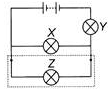If a third lamp Z is connected in parallel with lamp X, then what will happens to the brightness of the lamp V?

Detailed Solution for Olympiad Test: Electricity & Circuits - Question 1

The third lamp Z is connected parallel to X, so the current gets divided among X and Z. So both bulbs X and Z light dimmer and the bulb Y lights brighter than earlier because current through it increases.

Olympiad Test: Electricity & Circuits - Question 2

### Look at the given figure. It consists of a cell, a bulb with the two terminals X and Y and wires with ends P and 0; and S and R. The direction of current will be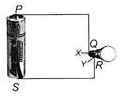Detailed Solution for Olympiad Test: Electricity & Circuits - Question 2

The direction of current will be PQRS because the current flows from positive terminal of the cell to the negative terminal

Olympiad Test: Electricity & Circuits - Question 3

### In which of the following circuits will the bulb glow?

Detailed Solution for Olympiad Test: Electricity & Circuits - Question 3

In figure (A), both ends of the bulb are connected to the negative terminal of the cell. So, bulb will not glow while in figure (B) and (C), insulating objects like string and rubber band are used. So, the circuit is incomplete and bulb will not glow. Figure (D) represents the complete circuit with proper connection. Hence, the bulb glows in figure (D).

Olympiad Test: Electricity & Circuits - Question 4

Which part of the bulb is an insulator?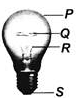Detailed Solution for Olympiad Test: Electricity & Circuits - Question 4

P is the glass cover, Q is the filament, R is the supporting wires, while S is the positive terminal of the bulb.

Olympiad Test: Electricity & Circuits - Question 5

To protect the user, part(s) ____ of the plug as shown in the figure must be made of electrical insulators.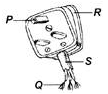Detailed Solution for Olympiad Test: Electricity & Circuits - Question 5

The plug cover R and connecting wires cover S should be made of electrical insulators.

Olympiad Test: Electricity & Circuits - Question 6

There is a mistake in the given classification table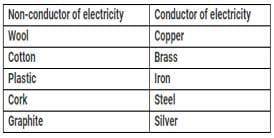Which of the following is placed incorrectly in the groups?

Detailed Solution for Olympiad Test: Electricity & Circuits - Question 6

Graphite is a conductor of electricity.

Olympiad Test: Electricity & Circuits - Question 7

The wire which carries away the current to the power house is called

Detailed Solution for Olympiad Test: Electricity & Circuits - Question 7

Reflection takes place whenever ray of light travels from a transparent medium towards an opaque medium . The opaque medium does not let the light pass through.

Olympiad Test: Electricity & Circuits - Question 8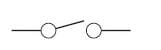What is the function of the component shown above?

Olympiad Test: Electricity & Circuits - Question 9

Which of the following statements are correct?
(i) Human body is a good conductor of electricity.
(ii) The air gap surrounding an electric circuit acts as a conductor.
(iii) Silver conducts electricity better than any other metal.
(iv) When electricity lights up a bulb, no heat is produced.

Detailed Solution for Olympiad Test: Electricity & Circuits - Question 9

Air is a bad conductor of electricity. When electricity lights up a bulb, heat is produced.

Olympiad Test: Electricity & Circuits - Question 10

Study the classification carefully and select the correct option for X, Y and Z.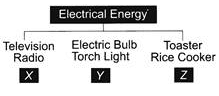Detailed Solution for Olympiad Test: Electricity & Circuits - Question 10

In X, the electrical energy is converted into sound energy. In Y, the electrical energy is converted into light energy. In Z, the electrical energy gets converted into heat energy. So, (C) is the correct option.

Olympiad Test: Electricity & Circuits - Question 11

"Do not handle electric wires with your bare hands. Wear rubber gloves," said Mr Gupta. Which of the following statements is not true about what Mr. Gupta commented?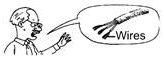Detailed Solution for Olympiad Test: Electricity & Circuits - Question 11

The rubber gloves are bad conductors of electricity. Hence, they prevent electric current to enter in our body and protect us from electric shocks.

Olympiad Test: Electricity & Circuits - Question 12

Nidhi has two bulbs connected across two cells in a simple circuit as shown. How can she make the bulbs glow dimmer?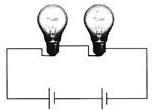Detailed Solution for Olympiad Test: Electricity & Circuits - Question 12

Replacing one cell with a piece of wire reduces the current in the circuit. Hence, the bulbs glow dimmer.

Olympiad Test: Electricity & Circuits - Question 13

Fuse is the most important safety device, used for protecting the circuits due to

Detailed Solution for Olympiad Test: Electricity & Circuits - Question 13

Fuse is a device used for short circuiting the circuit when overloading of current happens which helps to protect the electrical appliances.

Olympiad Test: Electricity & Circuits - Question 14

Direction: Observe the given figure and answer the following question.
The bulb will glow when a _____ is placed in between the probes.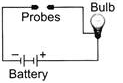Detailed Solution for Olympiad Test: Electricity & Circuits - Question 14

Key is a metallic object. When it is placed in between probes then current can easily flow in the circuit.

Olympiad Test: Electricity & Circuits - Question 15

Direction: Observe the given figure and answer the following question.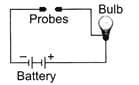Which of the following statements is correct regarding the maximum brightness of the bulb used in the circuit?

Detailed Solution for Olympiad Test: Electricity & Circuits - Question 15

Brightness of bulb can be increased by increasing the current through the bulb. This can be achieved by connecting a similar battery in the circuit.

Olympiad Test: Electricity & Circuits - Question 16

Three identical bulbs namely P, Q and R are connected to a battery as shown. What will happen when the circuit is closed?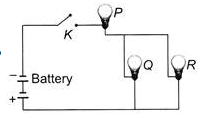Detailed Solution for Olympiad Test: Electricity & Circuits - Question 16

When the circuit is closed, an electric current starts flowing in the circuit from the positive terminal of battery. The current which passes through bulb P gets divided and flows in bulbs Q and R. As the brightness depends upon the current flowing through the bulb, so P will be bright but Q and R will be dim.

Olympiad Test: Electricity & Circuits - Question 17

In the given diagram, there are two switches S1 and S2 and two bulbs X and Y. If switch S1, is closed and switch S2 is open then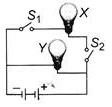Detailed Solution for Olympiad Test: Electricity & Circuits - Question 17

Since switch S2 is open and S1 is closed, the current will pass through Y but for bulb X, the circuit is incomplete. There is no complete path for current to flow for bulb X. Hence, only bulb Y will glow.

Olympiad Test: Electricity & Circuits - Question 18

Ravi connected three bulbs with the cells and a switch as shown. When switch is moved to ON position,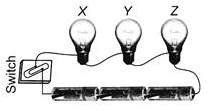Detailed Solution for Olympiad Test: Electricity & Circuits - Question 18

Current will flow positive to negative terminal, so all bulbs will glow simultaneously.

Olympiad Test: Electricity & Circuits - Question 19

Manu connected three bulbs to a cell as shown in figure. She found that filament of bulb is broken. Which of the following statements is correct?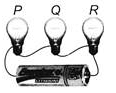Detailed Solution for Olympiad Test: Electricity & Circuits - Question 19

Bulb Q is fused because its filament is broken and as it is fused the circuit is broken. So none of the bulb will glow.

Olympiad Test: Electricity & Circuits - Question 20

Given diagram is a torch containing cells. The torch is not glowing when the switch is ON. Now, it is opened to know the reasons. Which of the following statements are correct regarding the reasons?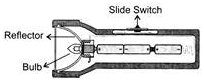(i) Bulb of the torch is fused.
(ii) Cells are not connected properly.
(iii) The metal parts of the torch do not make proper contact with the cell.

Detailed Solution for Olympiad Test: Electricity & Circuits - Question 20

The torch is not glowing because either its bulb is fused or cells are connected wrongly or metal spring of torch does not make proper contact with the cell.

## Science Class 6

113 videos|173 docs|48 tests
 Use Code STAYHOME200 and get INR 200 additional OFF Use Coupon Code
Information about Olympiad Test: Electricity & Circuits Page
In this test you can find the Exam questions for Olympiad Test: Electricity & Circuits solved & explained in the simplest way possible. Besides giving Questions and answers for Olympiad Test: Electricity & Circuits, EduRev gives you an ample number of Online tests for practice

## Science Class 6

113 videos|173 docs|48 tests